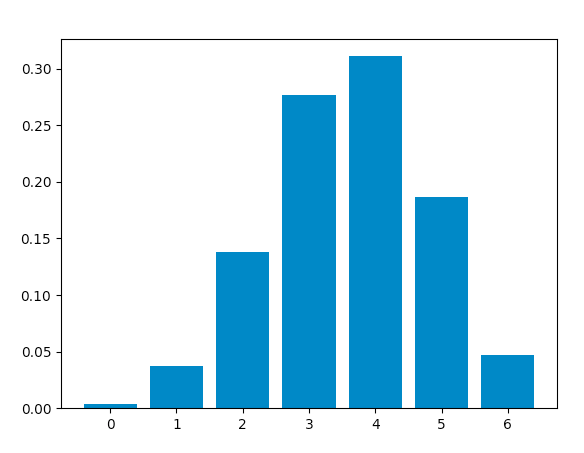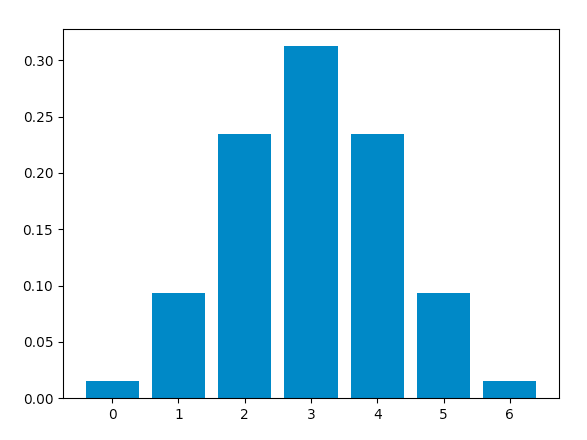Related Articles

# Python – Binomial Distribution

• Difficulty Level : Easy
• Last Updated : 16 Jul, 2020

Binomial distribution is a probability distribution that summarises the likelihood that a variable will take one of two independent values under a given set of parameters. The distribution is obtained by performing a number of Bernoulli trials.

A Bernoulli trial is assumed to meet each of these criteria :

• There must be only 2 possible outcomes.
• Each outcome has a fixed probability of occurring. A success has the probability of p, and a failure has the probability of 1 – p.
• Each trial is completely independent of all others.

The binomial random variable represents the number of successes(r) in n successive independent trials of a Bernoulli experiment.

Probability of achieving r success and n-r failure is :The number of ways we can achieve r successes is :Hence, the probability mass function(pmf), which is the total probability of achieving r success and n-r failure is :An example illustrating the distribution :

Consider a random experiment of tossing a biased coin 6 times where the probability of getting a head is 0.6. If ‘getting a head’ is considered as ‘success’ then, the binomial distribution table will contain the probability of r successes for each possible value of r.

This distribution has a mean equal to np and a variance of np(1-p)

Using Python to obtain the distribution :
Now, we will use Python to analyse the distribution(using SciPy) and plot the graph(using Matplotlib).
Modules required :

• SciPy:
SciPy is an Open Source Python library, used in mathematics, engineering, scientific and technical computing.

Installation :

pip install scipy

• Matplotlib:
Matplotlib is a comprehensive Python library for plotting static and interactive graphs and visualisations.

Installation :

pip install matplotlib


The scipy.stats module contains various functions for statistical calculations and tests. The stats() function of the scipy.stats.binom module can be used to calculate a binomial distribution using the values of n and p.

Syntax : scipy.stats.binom.stats(n, p)

It returns a tuple containing the mean and variance of the distribution in that order.

scipy.stats.binom.pmf() function is used to obtain the probability mass function for a certain value of r, n and p. We can obtain the distribution by passing all possible values of r(0 to n).

Syntax : scipy.stats.binom.pmf(r, n, p)

Calculating distribution table :

Approach :

• Define n and p.
• Define a list of values of r from 0 to n.
• Get mean and variance.
• For each r, calculate the pmf and store in a list.

Code :

 from scipy.stats import binom# setting the values# of n and pn = 6p = 0.6# defining the list of r valuesr_values = list(range(n + 1))# obtaining the mean and variance mean, var = binom.stats(n, p)# list of pmf valuesdist = [binom.pmf(r, n, p) for r in r_values ]# printing the tableprint("r\tp(r)")for i in range(n + 1):    print(str(r_values[i]) + "\t" + str(dist[i]))# printing mean and varianceprint("mean = "+str(mean))print("variance = "+str(var))

Output :

r    p(r)
0    0.004096000000000002
1    0.03686400000000005
2    0.13824000000000003
3    0.2764800000000001
4    0.31104
5    0.18662400000000007
6    0.04665599999999999
mean = 3.5999999999999996
variance = 1.44


Code: Plotting the graph using matplotlib.pyplot.bar() function to plot vertical bars.

 from scipy.stats import binomimport matplotlib.pyplot as plt# setting the values# of n and pn = 6p = 0.6# defining list of r valuesr_values = list(range(n + 1))# list of pmf valuesdist = [binom.pmf(r, n, p) for r in r_values ]# plotting the graph plt.bar(r_values, dist)plt.show()

Output :When success and failure are equally likely, the binomial distribution is a normal distribution. Hence, changing the value of p to 0.5, we obtain this graph, which is identical to a normal distribution plot :Attention geek! Strengthen your foundations with the Python Programming Foundation Course and learn the basics.

To begin with, your interview preparations Enhance your Data Structures concepts with the Python DS Course. And to begin with your Machine Learning Journey, join the Machine Learning – Basic Level Course

My Personal Notes arrow_drop_up